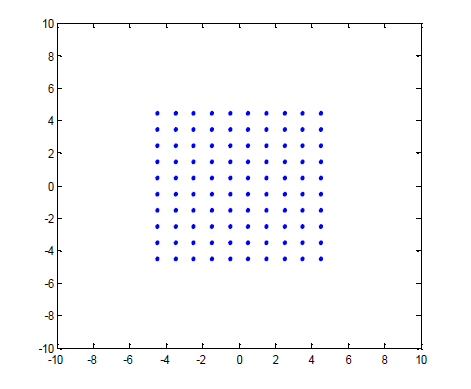## 问题背景## 信息量——方差/协方差矩阵

• 样本均值：$\operatorname{Mean}(\mathbf{x})=\frac{1}{n} \sum_{i=1}^n x_i=\frac{1}{n} \mathbf{x}^{\mathrm{T}} \mathbf{1}=\bar{x}$
• 样本方差：$\operatorname{Var}(\mathbf{x})=\frac{1}{n} \sum_{i=1}^n\left(x_i-\bar{x}\right)^2=\frac{1}{n}\|\tilde{\mathbf{x}}\|^2$
• 样本标准差：$\operatorname{S}(\mathbf{x})=\sqrt{\operatorname{Var}(\mathbf{x})}$
• 样本协方差：$\operatorname{Cov}(\mathbf{x}, \mathbf{y})=\frac{1}{n} \sum_{i=1}^n\left(x_i-\bar{x}\right)\left(y_i-\bar{y}\right)=\frac{1}{n} \tilde{\mathbf{x}}^{\mathrm{T}} \tilde{\mathbf{y}}$
• 样本相关系数：$r(\mathbf{x}, \mathbf{y})=\frac{\operatorname{Cov}(\mathbf{x}, \mathbf{y})}{S(\mathbf{x}) S(\mathbf{y})}$

$$\tilde{\mathbf{x}}=\left[\begin{array}{c} x_1-\bar{x} \\ x_2-\bar{x} \\ \vdots \\ x_n-\bar{x} \end{array}\right], \tilde{\mathbf{y}}=\left[\begin{array}{c} y_1-\bar{y} \\ y_2-\bar{y} \\ \vdots \\ y_n-\bar{y} \end{array}\right]$$

### 协方差矩阵与相关系数矩阵

$$\Sigma=\left[\begin{array}{cccc} \operatorname{cov}\left(\mathrm{x}_1, \mathrm{x}_1\right) & \operatorname{cov}\left(\mathrm{x}_1, \mathrm{x}_2\right) & \cdots & \operatorname{cov}\left(\mathrm{x}_1, \mathrm{x}_n\right) \\ \operatorname{cov}\left(\mathrm{x}_2, \mathrm{x}_1\right) & \operatorname{cov}\left(\mathrm{x}_2, \mathrm{x}_2\right) & \cdots & \operatorname{cov}\left(\mathrm{x}_2, \mathrm{x}_n\right) \\ \vdots & \vdots & \ddots & \vdots \\ \operatorname{cov}\left(\mathrm{x}_n, \mathrm{x}_1\right) & \operatorname{cov}\left(\mathrm{x}_n, \mathrm{x}_2\right) & \cdots & \operatorname{cov}\left(\mathrm{x}_n, \mathrm{x}_n\right) \end{array}\right]$$

$$\mathrm{C}=\left[\begin{array}{cccc} 1 & \frac{\operatorname{cov}\left(\mathrm{x}_1, \mathrm{x}_2\right)}{\sqrt{\operatorname{cov}\left(\mathrm{x}_1, \mathrm{x}_1\right)} \sqrt{\operatorname{cov}\left(\mathrm{x}_2, \mathrm{x}_2\right)}} & \cdots & \frac{\operatorname{cov}\left(\mathrm{x}_1, \mathrm{x}_n\right)}{\sqrt{\operatorname{cov}\left(\mathrm{x}_1, \mathrm{x}_1\right)} \sqrt{\operatorname{cov}\left(\mathrm{x}_n, \mathrm{x}_n\right)}} \\ \frac{\operatorname{cov}\left(\mathrm{x}_2, \mathrm{x}_1\right)}{\sqrt{\operatorname{cov}\left(\mathrm{x}_1, \mathrm{x}_1\right)} \sqrt{\operatorname{cov}\left(\mathrm{x}_2, \mathrm{x}_2\right)}} & \cdots & \frac{\operatorname{cov}\left(\mathrm{x}_2, \mathrm{x}_n\right)}{\sqrt{\operatorname{cov}\left(\mathrm{x}_2, \mathrm{x}_2\right)} \sqrt{\operatorname{cov}\left(\mathrm{x}_n, \mathrm{x}_n\right)}} \\ \vdots & 1 & \ddots & \vdots \\ \frac{\operatorname{cov}\left(\mathrm{x}_n, \mathrm{x}_1\right)}{\sqrt{\operatorname{cov}\left(\mathrm{x}_1, \mathrm{x}_1\right)} \sqrt{\operatorname{cov}\left(\mathrm{x}_n, \mathrm{x}_n\right)}} & \frac{\operatorname{cov}\left(\mathrm{x}_n, \mathrm{x}_2\right)}{\sqrt{\operatorname{cov}\left(\mathrm{x}_2, \mathrm{x}_2\right)} \sqrt{\operatorname{cov}\left(\mathrm{x}_n, \mathrm{x}_n\right)}} & \cdots & 1 \end{array}\right]$$

## 数据中心化

$$\mathbf{X}=\left[\begin{array}{c} X_1 \\ \vdots \\ X_L \end{array}\right]=\left[\mathbf{x}_1, \mathbf{x}_2, \cdots, \mathbf{x}_N\right]$$

$$\boldsymbol{\mu}=\frac{1}{N}\left[\begin{array}{c} X_1 \mathbf{1}_N \\ \vdots \\ X_L \mathbf{1}_N \end{array}\right]=\frac{1}{N} \mathbf{X} \mathbf{1}_N=\frac{1}{N} \sum_{i=1}^N \mathbf{x}_i$$

\begin{aligned} \bar{\mathbf{X}}&=\left[\mathbf{x}_1-\boldsymbol{\mu}, \mathbf{x}_2-\boldsymbol{\mu}, \cdots, \mathbf{x}_N-\boldsymbol{\mu}\right] \\ &=\mathbf{X}-\boldsymbol{\mu} \mathbf{1}_N^{\mathrm{T}} \\ &=\mathbf{X}-\frac{1}{N} \mathbf{X} \mathbf{1}_N \mathbf{1}_N^{\mathrm{T}} \\ &=\mathbf{X}\left(\mathbf{I}-\frac{1}{N} \mathbf{1}_N \mathbf{1}_N^{\mathrm{T}}\right) \end{aligned}

\begin{aligned} \mathbf{P}_{\mathbf{1}_N}&= \mathbf{1}_N\mathbf{1}_{N}^\# \\ &=\mathbf{1}_N(\mathbf{1}_N^T\mathbf{1}_N)^{-1}\mathbf{1}_N^T \\ &=\frac{1}{N}\mathbf{1}_N\mathbf{1}_N^T \end{aligned}

$$\mathbf{P}_{\mathbf{1}_N}^\perp = \mathbf{I}-\frac{1}{N} \mathbf{1}_N \mathbf{1}_N^{\mathrm{T}}$$

$$\mathbf{X}\left(\mathbf{I}-\frac{1}{N} \mathbf{1}_N \mathbf{1}_N^{\mathrm{T}}\right)=\left(\mathbf{P}_{\mathbf{1}_N}^\perp\mathbf{X}^T \right)^T$$

## 协方差矩阵的计算

\begin{aligned} \Sigma&=\left[\begin{array}{ccc} \operatorname{cov}\left(X_1, X_1\right) & \cdots & \operatorname{cov}\left(X_1, X_L\right) \\ \vdots & \ddots & \vdots \\ \operatorname{cov}\left(X_L, X_1\right) & \cdots & \operatorname{cov}\left(X_L, X_L\right) \end{array}\right] \\ &=\left[\begin{array}{ccc} \frac{1}{N} X_1 X_1^{\mathrm{T}} & \cdots & \frac{1}{N} X_1 X_L^{\mathrm{T}} \\ \vdots & \ddots & \vdots \\ \frac{1}{N} X_L X_1^{\mathrm{T}} & \cdots & \frac{1}{N} X_L X_L^{\mathrm{T}} \end{array}\right] \\ &=\frac{1}{N}\left[\begin{array}{c} X_1 \\ \vdots \\ X_L \end{array}\right]\left[X_1^{\mathrm{T}}, \cdots, X_L^{\mathrm{T}}\right] \\ &=\frac{1}{N} \mathbf{X X}^{\mathrm{T}} \\ &=\frac{1}{N}\left[\mathbf{x}_1, \cdots, \mathbf{x}_N\right]\left[\begin{array}{c} \mathbf{x}_1^{\mathrm{T}} \\ \vdots \\ \mathbf{x}_N^{\mathrm{T}} \end{array}\right] \\ &=\frac{1}{N} \sum_{\mathrm{i}=1}^N \mathrm{x}_{\mathrm{i}} \mathrm{x}_{\mathrm{i}}^{\mathrm{T}} \end{aligned}

## 任意方向方差的计算

\begin{aligned} \mathrm{Var}(\mathbf{u}^T\mathbf{X})&=\frac{1}{N}\|\mathbf{u}^T\mathbf{X}\|^2 \\ &=\frac{1}{N}\mathbf{u}^T\mathbf{X}\mathbf{X}^T\mathbf{u} \\ &=\mathbf{u}^T \Sigma \mathbf{u} \end{aligned}

## 主成分分析

$$\left\{\begin{array}{l} \max _{\mathbf{u}} \mathbf{u}^T \Sigma \mathbf{u} \\ \mathbf{u}^T \mathbf{u}=1 \end{array}\right.$$

$$F(\mathbf{u},\lambda)=\frac{1}{2}\mathbf{u}^T \Sigma \mathbf{u}+\frac{1}{2}\lambda(1-\mathbf{u}^T \mathbf{u})$$

$$\frac{\partial F}{\partial\mathbf{u}}=\Sigma \mathbf{u}-\lambda \mathbf{u}$$

$$\Sigma \mathbf{u}=\lambda \mathbf{u}$$

## 主成分分析的步骤

1. 数据中心化：$\boldsymbol{\mu}=\frac{1}{N} \mathbf{X 1}^T \quad \mathbf{X}=\mathbf{X}-\boldsymbol{\mu} \mathbf{1}^T$
2. 计算协方差矩阵：$\Sigma=\frac{1}{N}\mathbf{X}\mathbf{X}^T$
3. 计算协方差矩阵的特征值和特征向量：$\mathbf{\Sigma}\mathbf{U}=\mathbf{U}\mathbf{\Lambda}$
4. 主成分变换：$\mathbf{Y}=\mathbf{U}^T\mathbf{X}$

$$\mathbf{u}^T\Sigma \mathbf{u}=\mathbf{u}^T\lambda \mathbf{u}=\lambda\mathbf{u}^T \mathbf{u}=\lambda$$

## PCA几何解释

$$\operatorname{Var}\left(\mathbf{u}^T \mathbf{X}\right)=\mathbf{u}^T \Sigma \mathbf{u}=\frac{1}{N} \mathbf{u}^T \mathbf{X} \mathbf{X}^T \mathbf{u}=\frac{1}{N}\left\|\mathbf{u}^T \mathbf{X}\right\|^2$$

$$\mathbf{u}^{\mathrm{T}} \mathbf{X}=\left[\mathbf{u}^{\mathrm{T}} \mathbf{x}_1, \mathbf{u}^{\mathrm{T}} \mathbf{x}_2, \cdots, \mathbf{u}^{\mathrm{T}} \mathbf{x}_N\right]=u_1 X_1+u_2 X_2+\cdots+u_L X_L$$

$$\mathbf{y}=(\mathbf{u}^T\mathbf{X})^T=\mathbf{X}^T\mathbf{u}$$

$$\mathbf{y}^T \mathbf{X}^T\left(\mathbf{X} \mathbf{X}^T\right)^{-1}\left(\mathbf{X} \mathbf{X}^T\right)^{-1} \mathbf{X} \mathbf{y}=1$$

$$f(\mathbf{y})=\mathbf{y}^T \mathbf{X}^T\left(\mathbf{X X}^T\right)^{-1}\left(\mathbf{X} \mathbf{X}^T\right)^{-1} \mathbf{X} \mathbf{y}-1=0$$

## PCA的进一步解释

$$\mathbf{Q}=\left[\begin{array}{llll} \mathbf{q}_1 & \mathbf{q}_2 & \cdots & \mathbf{q}_s \end{array}\right]$$

$$\mathbf{P}_{\mathbf{Q}}=\mathbf{Q} \mathbf{Q}^{\#}=\mathbf{Q}\left(\mathbf{Q}^{\mathrm{T}} \mathbf{Q}\right)^{-1} \mathbf{Q}^{\mathrm{T}}=\mathbf{Q} \mathbf{Q}^{\mathrm{T}}$$

$$\mathbf{Y}=\mathbf{P}_{\mathbf{Q}} \mathbf{X}=\mathbf{Q Q}^{\mathrm{T}} \mathbf{X}$$

$$\left\{\begin{array}{l} \min _{\mathbf{Q}}\left\|\left(\mathbf{I}_p-\mathbf{Q} \mathbf{Q}^{\mathrm{T}}\right) \mathbf{X}\right\|_F^2 \\ \text { s.t } \mathbf{Q}^{\mathrm{T}} \mathbf{Q}=\mathbf{I}_s \end{array}\right.$$

\begin{aligned} \left\|\left(\mathbf{I}_p-\mathbf{Q Q}^{\mathrm{T}}\right) \mathbf{X}\right\|_F^2&=\operatorname{trace}\left(\mathbf{X}^{\mathrm{T}}\left(\mathbf{I}_p-\mathbf{Q Q}^{\mathrm{T}}\right)\left(\mathbf{I}_p-\mathbf{Q} \mathbf{Q}^{\mathrm{T}}\right) \mathbf{X}\right) \\ &=\operatorname{trace}\left(\mathbf{X}^{\mathrm{T}}\left(\mathbf{I}_p-\mathbf{Q} \mathbf{Q}^{\mathrm{T}}\right) \mathbf{X}\right) \\ &=\operatorname{trace}\left(\mathbf{X}^{\mathrm{T}} \mathbf{X}\right)-\operatorname{trace}\left(\mathbf{Q}^{\mathrm{T}} \mathbf{X} \mathbf{X}^{\mathrm{T}} \mathbf{Q}\right) \end{aligned}

$$\left\{\begin{array}{l} \min _{\mathbf{Q}} \operatorname{trace}\left(\mathbf{Q}^{\mathrm{T}} \mathbf{X} \mathbf{X}^{\mathrm{T}} \mathbf{Q}\right) \\ \text { s.t } \mathbf{Q}^{\mathrm{T}} \mathbf{Q}=\mathbf{I}_s \end{array}\right.$$

## PCA的概率解释

$$l(\mathbf{Q})=\ln L(\mathbf{Q})=\sum_{i, j} \ln \frac{1}{\sqrt{2 \pi} \sigma}-\frac{1}{2 \sigma^2} \sum_{i, j} e_{i j}^2$$

$$\sum_{i, j} e_{i j}^2=\left\|\left(\mathbf{I}_p-\mathbf{Q Q}^{\mathrm{T}}\right) \mathbf{X}\right\|_F^2$$

## 发送评论编辑评论

|´・ω・)ノ
ヾ(≧∇≦*)ゝ
(☆ω☆)
（╯‵□′）╯︵┴─┴
￣﹃￣
(/ω＼)
∠( ᐛ 」∠)＿
(๑•̀ㅁ•́ฅ)
→_→
୧(๑•̀⌄•́๑)૭
٩(ˊᗜˋ*)و
(ノ°ο°)ノ
(´இ皿இ｀)
⌇●﹏●⌇
(ฅ´ωฅ)
(╯°A°)╯︵○○○
φ(￣∇￣o)
ヾ(´･ ･｀｡)ノ"
( ง ᵒ̌皿ᵒ̌)ง⁼³₌₃
(ó﹏ò｡)
Σ(っ °Д °;)っ
( ,,´･ω･)ﾉ"(´っω･｀｡)
╮(╯▽╰)╭
o(*////▽////*)q
＞﹏＜
( ๑´•ω•) "(ㆆᴗㆆ)
( ゜- ゜)つロ
_(:з」∠)_
（⌒▽⌒）
（￣▽￣）
(=・ω・=)
(*°▽°*)八(*°▽°*)♪
✿ヽ(°▽°)ノ✿
(¦3【▓▓】
눈_눈
(ಡωಡ)
_(≧∇≦」∠)_
━━━∑(ﾟ□ﾟ*川━
(｀・ω・´)
(￣3￣)
✧(≖ ◡ ≖✿)
(･∀･)
(〜￣△￣)〜
→_→
(°∀°)ﾉ
╮(￣▽￣)╭
( ´_ゝ｀)
←_←
(;¬_¬)
(ﾟДﾟ≡ﾟдﾟ)!?
( ´･･)ﾉ(._.)
Σ(ﾟдﾟ;)
Σ( ￣□￣||)<
(´；ω；)
（/TДT)/
(^・ω・^)
(｡･ω･｡)
(●￣(ｴ)￣●)
ε=ε=(ノ≧∇≦)ノ
(´･_･)
(-_-#)
（￣へ￣）
(￣ε(#￣) Σ
(╯°口°)╯(┴—┴
ヽ(Д´)ﾉ
("▔□▔)/
(º﹃º )
(๑>؂<๑）
｡ﾟ(ﾟ´Д｀)ﾟ｡
(∂ω∂)
(┯_┯)
(・ω< )★
( ๑ˊ•̥▵•)੭₎₎
¥ㄟ(´･ᴗ･)ノ¥
Σ_(꒪ཀ꒪」∠)_
٩(๛ ˘ ³˘)۶❤
(๑‾᷅^‾᷅๑)

Emoji

tv_小电视